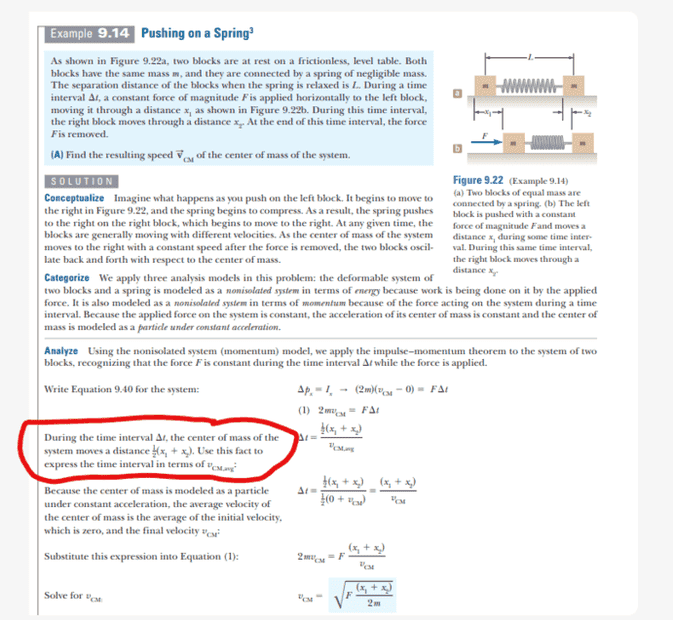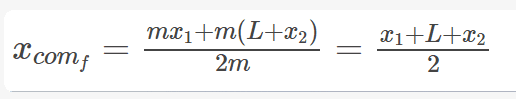# Measuring COM of system

• String theory guy
String theory guy
Homework Statement
Why must you measure the COM of a system from the origin before and after a collision?
Relevant Equations
COM formula
For example, in the problem below, if the center of mass is chosen to be measured initially at the center of the left mass, then it must be measured from the same position after the collision.This gives an initial COM of,and finial COM of,Which gives their change in center of mass of the system.

So why must I find the finial COM of the system with respect to the same place which I used to find the COM before the force was exerted on the system.

Homework Helper
Gold Member
2022 Award
Homework Statement:: Why must you measure the COM of a system from the origin before and after a collision?
Relevant Equations:: COM formula

For example, in the problem below, if the center of mass is chosen to be measured initially at the center of the left mass, then it must be measured from the same position after the collision.

View attachment 318103

This gives an initial COM of,

View attachment 318104

and finial COM of,

View attachment 318105

Which gives their change in center of mass of the system.

So why must I find the finial COM of the system with respect to the same place which I used to find the COM before the force was exerted on the system.
Because what we are trying to find is how far the CoM moved.

•String theory guy
ChiralSuperfields
Homework Statement:: Why must you measure the COM of a system from the origin before and after a collision?
Relevant Equations:: COM formula

For example, in the problem below, if the center of mass is chosen to be measured initially at the center of the left mass, then it must be measured from the same position after the collision.

View attachment 318103

This gives an initial COM of,

View attachment 318104

and finial COM of,

View attachment 318105

Which gives their change in center of mass of the system.

So why must I find the finial COM of the system with respect to the same place which I used to find the COM before the force was exerted on the system.
You can measure the center of mass from any position as long as you do it from the same place before any after the force. Hope that helps.

•String theory guy